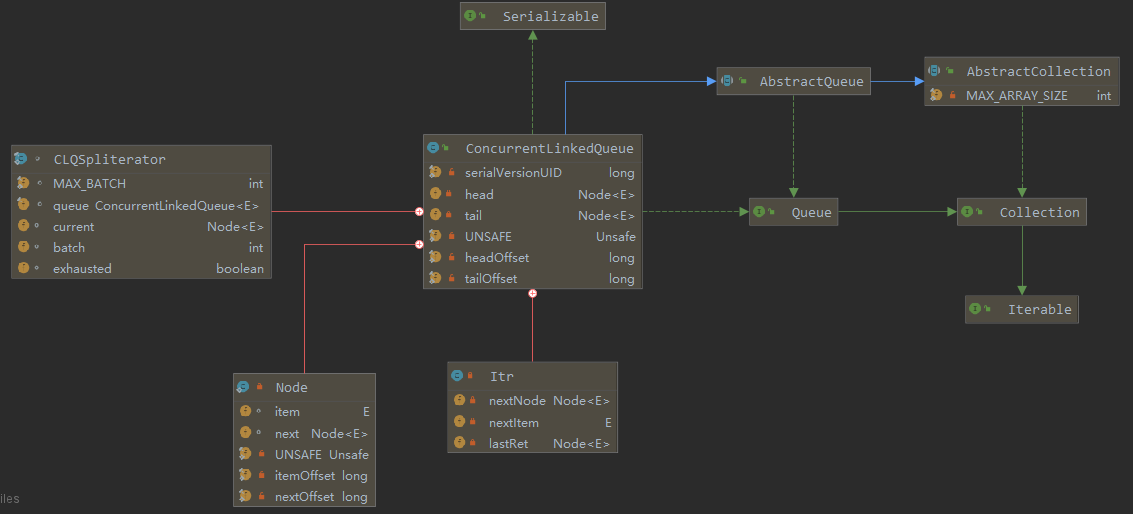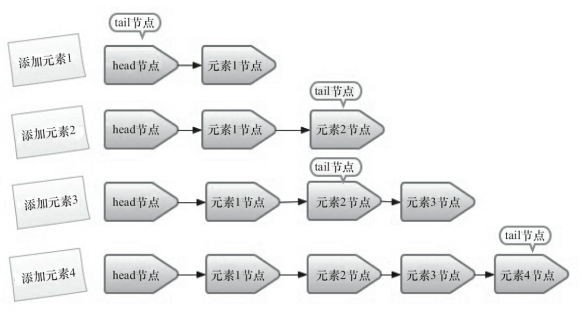Categories

I. INTRODUCTION

ConcurrentLinkedQueue is an unbounded thread-safe queue based on linked nodes, non-blocking, which uses FIFO rule sorting nodes, when we add an element when it is added to the tail of the queue; when we get an element, it returns the element head of the queue.

ConcurrentLinkedQueue non-blocking way to achieve thread-safe queue, it uses a “wait-free” algorithm (ie CAS algorithm) to achieve.ConcurrentLinkedQueue the head node and tail nodes, each node (Node) and a reference pointing to the next node element (item) a node (next) the composition, is between the node and the node by correlating the next, so as to constitute a list queue structure. The default case where the head node storage element is null, tail head node is equal to the node.

Second, the interpretation of the source

Now we have a head and a tail node, according to our usual thinking, head node that is head node, tail node that is the end node. Then, when the queue, the tail of the next node is set to newNode the tail newNode set; when the queue, the head node element returned, the next head to head node. Codes are as follows:

``````    public boolean offer(E e) {
if (e == null)
throw new NullPointerException();
Node n = new Node(e);
for (; ; ) {
Node t = tail;
if (t.casNext(null, n) && casTail(t, n)) {
return true;
}
}
}``````

Do not doubt your thinking, such an approach is entirely feasible.ConcurrentLinkedQueue want to read the source code, it is best to get to know the following characteristics:

next only the last element in the queue at any time is null

head and tail is not null (Sentinel node design)

head may not be the first element in the queue (head may be a pointing element has been removed)

tail is not the last element in the queue (tail.next may not be null)

Once the item becomes an element of null, it means that it is no longer valid elements in the queue, and will be deleted the next node pointer to itself.

– the queue

``````    public boolean offer(E e) {
// 1.
checkNotNull(e);
final Node newNode = new Node(e);

for (Node t = tail, p = t;;) {
Node q = p.next;
// 2.
if (q == null) {
// 3.
if (p.casNext(null, newNode)) {
// 4.
if (p != t)
casTail(t, newNode);
return true;
}
}
// 5.
else if (p == q)
p = (t != (t = tail)) ? t : head;
else
// 6.
p = (p != t && t != (t = tail)) ? t : q;
}
}``````

Queue element does not allow null, or throw a NullPointerException

p q == null description is the last element in the queue

CAS is set into the team newNode

t is a snapshot of the current thread read tail, p is the last element of the above CAS queue. When p! = Tail instructions to update the node t. If the CAS fails then the other thread tail that has been updated, and it does not matter.

p == q Under what circumstances do? Only when the p elements no longer in the queue, namely p == p.next. This time how to do it? To re-read snapshot of a tail t. If t is changed, the tail from the new node to continue (the same as the initial value of the attention here and set value for the cycle, indicating restarted, continue to try). If t does not change, indicating that the tail element has also been in the queue outside (because the queue is a first in first out), in which case we need to continue to traverse down from the head.

If p and t are equal, indicating that p is not the end node, a node so that p continues to move backward; if p and t are not equal, then the cycle has gone through at least two rounds (still not into the team), then re-read a tail to t, p = t (and initial values ​​for the same as above), it indicates start over again enqueued.

– the queue

``````    public E poll() {
for (;;) {
// 1.
for (Node h = head, p = h, q;;) {
E item = p.item;

// 2.
if (item != null && p.casItem(item, null)) {
// 3.
if (p != h)
updateHead(h, ((q = p.next) != null) ? q : p);
return item;
}
// 4.
else if ((q = p.next) == null) {
return null;
}
// 5.
else if (p == q)
// 6.
else
p = q;
}
}
}``````

As the head snapnode h, p is set to the initial head, q = null.

CAS item successfully set to null, indicates that the elements have been removed (note that this time the element has not really removed from the queue, p = p.next actions completed in updateHead in). item here! = null judgment is meaningless to try to avoid the CAS.

When p is not equal to H, described lags head node, to update the head node. If p.next node is not equal to null, put the head set p.next; if p.next == null, shows that p is the last node, no way, can only be placed on the head node of the p. updateHead will do an action – p = p.next, the lag p nodes officially removed from the queue.

Similar to the first 3:00 explanation, if p.next == null, it indicates that this is the last node, and you can only update the head is p-node, returns null.

P == q it will appear under what circumstances? That p == p.next. This is the case, suggesting that other threads completed ahead of schedule, p elements have been removed from the queue. This time to continue the cycle of little significance, so just start again, re-read head to a snapshot h, and then try to remove the element.

p moved back a (then q = p.next), continue to try to remove elements.

Three, API use

return value

method

Explanation

Inserted at the tail of the queue of the specified element

Appended to the end of the queue in the order set returned by the iterator

The queue contains a specified element

If the queue contains the central element, return true

Returns an iterator elements in this queue, the element start from scratch iteration

Retrieves, but does not remove the head of the queue, if the queue is empty, null is returned

Retrieve and delete the head of the queue, if the queue is empty or null

Removes the specified element from the queue in a single instance (if present)

Returns the number of elements in the queue

Queue turn into an array of the specified type

boolean add(E e) / offer(E e)